Next: Planck Radiation Law Up: Quantum Statistics Previous: Quantum-Mechanical Treatment of Ideal

# Derivation of van der Waals Equation of State

We shall now extend the analysis of the previous section to derive an approximate equation of state for a non-ideal gas. Let us focus attention on a single molecule. It seems reasonable to suppose that this molecule moves in an effective potential,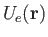, due to all the other molecules in the gas (which are assumed to remain unaffected by the presence of the molecule under consideration). Under these circumstances, the partition function of the gas reduces to that of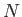independent particles, each with a kinetic energy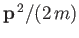, and a potential energy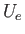.

Proceeding classically (except that the molecules are treated as indistinguishable, and the arbitrary parameter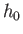is replaced by Planck's constant), we obtain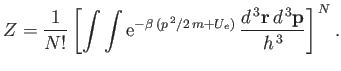(8.85)

(See Sections 7.7 and 8.10.) Thus,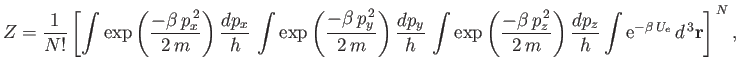(8.86)

which yields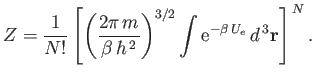(8.87)

(See Exercise 2.)

The remaining integral extends over the volume,, of the container. To make further progress, we note that there are regions in which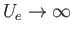because of the strong repulsion between molecules when they approach one another too closely. Thus, the integrand vanishes in these regions, which are assumed to have a total volume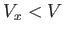. In the remaining volume,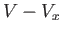, whereis assumed to vary relatively slowly with inter-molecular separation, we shall replaceby some effective constant average value,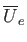. Thus, the previous equation becomes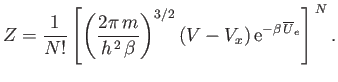(8.88)

It remains to estimate the values of the parametersand. The total mean potential energy of the molecules is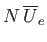. But, because there are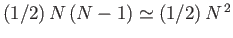pairs of molecules in the gas, it follows that the total mean potential energy is also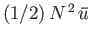, where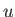is the potential energy of interaction between a given pair of molecules. Equating the different expressions for the total mean potential energy of the gas, we obtain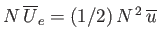, or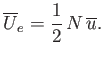(8.89)

To estimate the mean potential energy,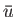, between a pair of molecules, let us make the simplistic assumption that the interaction potential takes the form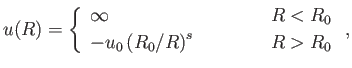(8.90)

where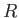is separation between the molecular centers,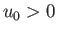, and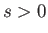. Thus, the molecules act as weakly-attracting hard spheres of radius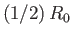. The choice of exponent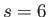is usually the most appropriate. (See Exercise 8.) Concentrating attention on a particular molecule, and making the crudest possible approximation, we shall say that another molecule is equally likely to be anywhere in the container that is a distancegreater than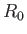from the given molecule. Thus, the probability of the distance lying betweenand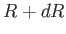is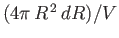, and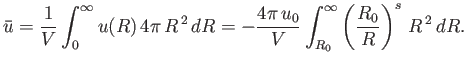(8.91)

Let us assume that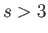: in other words, the potential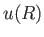falls off sufficiently rapidly for the previous integral to converge properly. Thus, Equation (8.89) becomes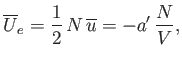(8.92)

where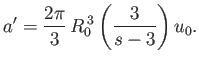(8.93)

By Equation (8.90), the distance of closest approach between molecules is. Thus, in each encounter between a pair of molecules, there is a volume excluded to one molecule, by virtue of the presence of the other molecule, that is equal to the volume of a sphere of radius. Because there arepairs of molecules, the total excluded volume is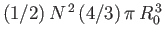. But, for the sake of consistency, this volume must be equal to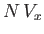, becausewas understood to be the excluded volume per molecule. Thus, it follows that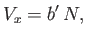(8.94)

where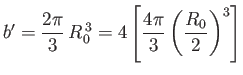(8.95)

is four times the volume of a hard-sphere molecule.

This completes our crude evaluation of the partition function. The equation of state of the gas is given by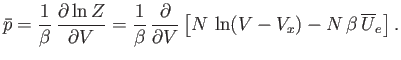(8.96)

Making use of Equations (8.92) and (8.94), we obtain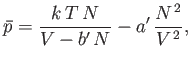(8.97)

or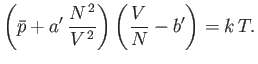(8.98)

Now,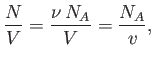(8.99)

where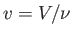is the molar volume. Thus, our approximate equation of state becomes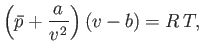(8.100)

where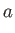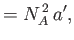(8.101)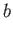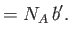(8.102)

Of course, Equation (8.100) is identical to the well-known van der Waals equation of state. (See Section 6.15.) Our analysis allows us to relate the constants,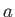and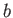, appearing in this equation of state, to the inter-molecular potential.Next: Planck Radiation Law Up: Quantum Statistics Previous: Quantum-Mechanical Treatment of Ideal
Richard Fitzpatrick 2016-01-25